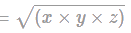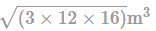The areas of three adjacent faces of a cuboi...
The areas of three adjacent faces of a cuboidal tank are 3 m2,12 m2 and 16 m2. The capacity of the tank, in litres, is:
The areas of three adjacent faces of a cuboidal tank are 3 m2,12 m2 a...
Given
Areas of 3 adjacent faces of cuboidal tank = 3 m2,12 m2,16 m2
As we know,
1 m3 = 1000 Litres
If areas of 3 adjacent faces of a cuboid are x,y and z respectively, then
Volume of cuboid =Areas of 3 adjacent faces of cuboidal tank = 3 m2, 12 m2, 16 m2
Volume of cuboid =Capacity of tank =Capacity of tank = 24 m3
1 m3=1000 Litres
Capacity of tank = 24 × 1000 lites
Capacity of tank = 24000 litres
∴ The capacity of the tank, (in litres) is 24000 litres.
Hence, the correct answer is 24000.
 1 Crore+ students have signed up on EduRev. Have you?
1 Crore+ students have signed up on EduRev. Have you?

### Learn this topic in detailIPMAT Mock Test - 9 (New Pattern) 100 Ques | 90 MinsMore from Related Course Quantitative Aptitude (Quant)View courses related to this question

### Quick links for CAT exam850+
Video Lectures2500+
Revision Notes600+
Online Tests10,000+
Doubts Solved
The areas of three adjacent faces of a cuboidal tank are 3 m2,12 m2 and 16 m2. The capacity of the tank, in litres, is:Correct answer is '24000'. Can you explain this answer?
Question Description(Scan QR code)